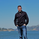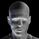2621瀏覽
Summary:

Calculate the distance of the price to a moving average. Also be able to identify if the average distance is decreasing or increasing based on signal line.

Details
Length: The moving average length to measure against.
Source: The price input source use to measure the distance from.
Signal Length: The average of distance between Source and MA.

If the average is increasing the color of the signal line will be green and if it is decreasing then it will be red.

Quadrant top left shows distance and signal line from the MA in terms of dollar amount.

Quadrant top left shows distance and signal line from the MA in terms of percentage.

Quadrant bottom left shows distance from MA in terms of dollar amount and signal line from the MA in terms of percentage. This is perhaps the most important measure of strength and also can be an indicator for overbought or oversold levels.

Quadrant bottom right shows distance from MA in terms of percentage and signal line from the MA in terms of dollar amount.

## 評論Simple yet awesome. I'm going to borrow some of your code for my indicator if you don't mind :)Very nice. How can I calculate if two emas distance is below a certain percentage? Basically, I am trying to find the
emaDifference = ema(src, 5) - ema(src, 100)
then add an indicator if the percentage is low so it shows if the emas are too close to each other up or down market.
Thanks.BertMan
@BertMan, You can switch (mark as checked) on 'Show Dist as %'. So now Plot #1 shows all values in %. Then create an alert on the indicator's Plot #1 value to be 'Less than' the % that you want it to be lower.FonF0n
@FonF0n, You can change the 'Source' to 'ohlc4' to consider the average of open-high-low-close or 'hl2' i.e. average of high & low. Is that what you meant to ask? Thanks for the thumbs up.